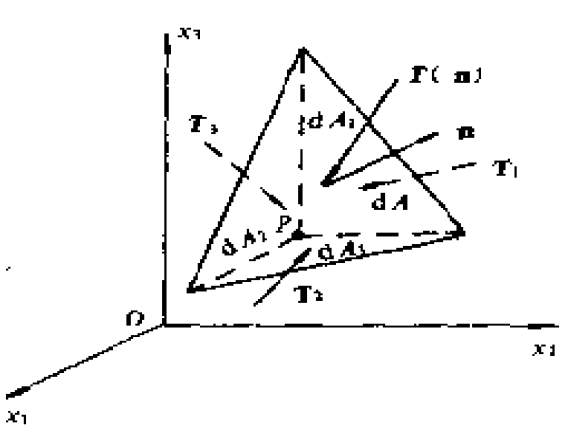# 流体力学引论

## 流体力学引论

#### 应力张量

T i ( n ) = σ 1 i n 1 + σ 2 i n 2 + σ 3 i n 3 T_{i}(\mathbf{n})=\sigma_{1 i} n_{1}+\sigma_{2i} n_{2}+\sigma_{3 i} n_{3}A = ( σ 11 σ 12 σ 13 σ 21 σ 22 σ 23 σ 31 σ 32 σ 33 ) A=\left(\begin{array}{lll}{\sigma_{11}} & {\sigma_{12}} & {\sigma_{13}} \\ {\sigma_{21}} & {\sigma_{22}} & {\sigma_{23}} \\ {\sigma_{31}} & {\sigma_{32}} & {\sigma_{33}}\end{array}\right)

A A 的某个特征向量和面元法向共线时，此时面元应力 T ( n ) \mathbf{T(n)} 和法向也是共线的。事实上，
T ( n ) = A n = λ n \mathbf{T(n)}=A\mathbf{n}=\lambda \mathbf{n}

p ˉ = − 1 3 trace ( A ) \bar p=-\frac{1}{3}\text{trace}(A)

#### 热力学基础

f ( p , ρ , T ) = 0 f(p, \rho, T)=0

E 2 − E 1 = ∫ 1 2 ( δ Q + δ W ) E_{2}-E_{1}=\int_{1}^{2}(\delta Q+\delta W)

h = ε + p v = ε + p / ρ h=\varepsilon+p v=\varepsilon+p / \rho

S 2 − S 1 = ∫ 1 2 ( δ Q T ) R S_{2}-S_{1}=\int_{1}^{2}\left(\frac{\delta Q}{T}\right)_{R}

K = ρ c 2 {K}=\rho c^{2}
K K 越大，表示流体越难被压缩。

03-14
12-2712-23
10-299208
06-10202
03-198995
06-113348
11-177万+
01-195万+
11-094422
09-296035
08-034501
08-276391
08-142989
11-15813
11-129010